# CCD Continuous Counter-Current Decantation Cyanidation Flowsheet

PROCESS FLOWSHEET DESCRIPTION: Continuous counter-current decantation cyanidation flowsheet.

ORE TREATED: Gold and silver ores amenable to the cyanidation process and where economics justify plant outlay.

ADVANTAGES: By producing the precious metals in bullion form, the highest net return is realized on many gold and silver ores. Where the tonnage available for treatment justifies the capital outlay, and where this process gives high recovery with satisfactory chemical consumption, this flowsheet is recommended.

PROCESSING COMMENTS: The counter-current decantation washing circuit for removing the solutions carrying the dissolved precious metals is rapidly supplanting other methods. In this circuit, wash water and barren solution are added in the last thickener units and flow toward the head of the plant, becoming enriched and are finally passed to the clarification and precipitation units where the precious metals are precipitated and recovered. The ore pulp (carrying the dissolved gold and silver) flows in the opposite direction, or counter-current, and becomes depleted in soluble value until finally discharged as a tailing with little or no contained soluble values.

Compared with the use of filters, lower capital cost, lower power cost, and lower labor cost more than offset the slightly higher loss in dissolved values, and improve net operating profits.

The Positive Type Washing Tray Thickener, requiring a small fraction of former floor and building space, is now replacing individual thickeners and allows the use of this attractive circuit in colder sections where previously building and heating costs prevented this circuit from being considered.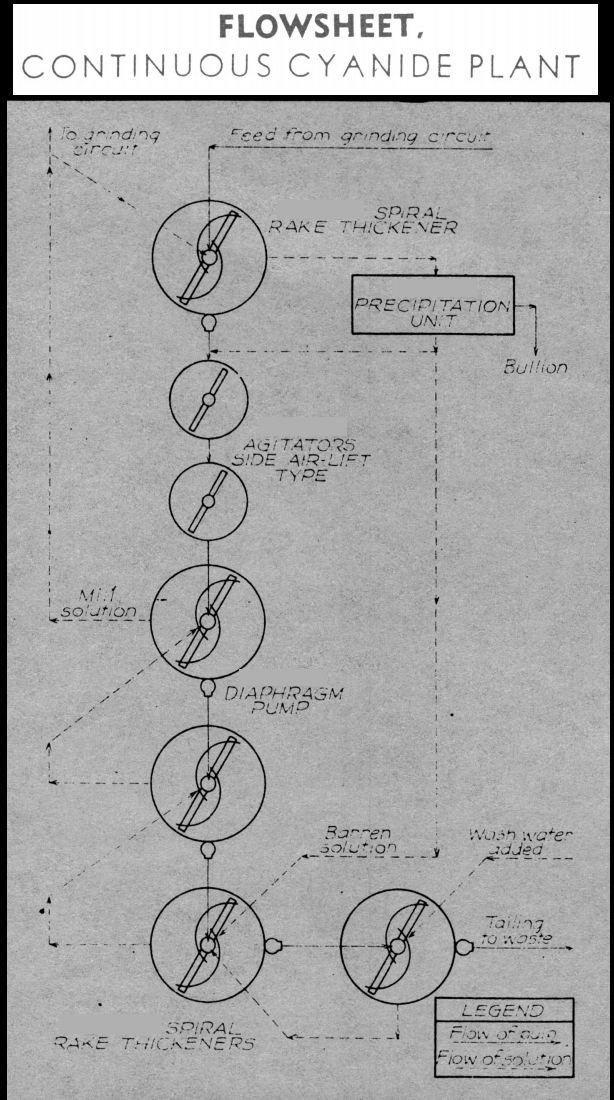## Continuous Counter Current Decantation Calculations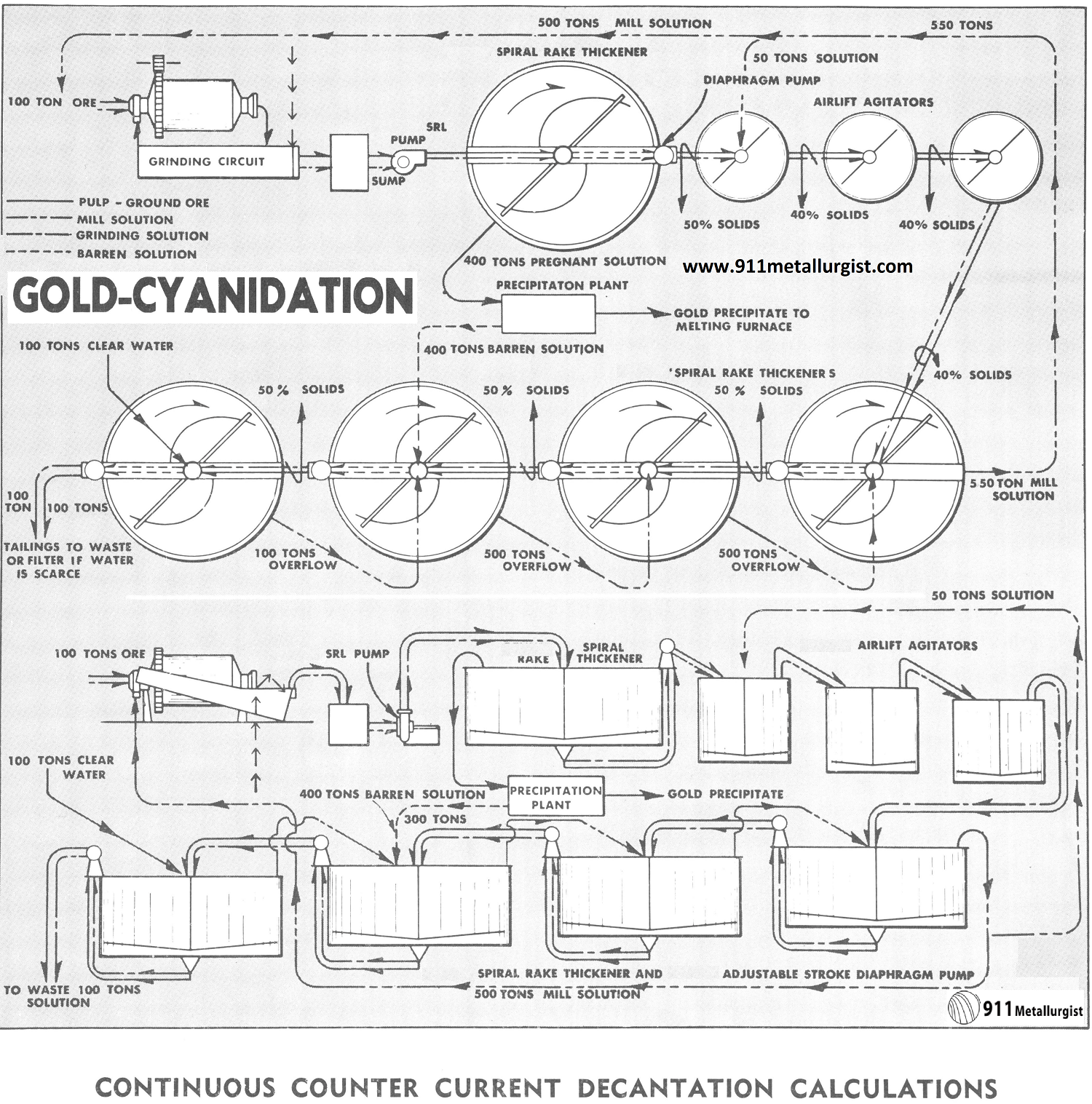### The Problem with Continuous counter current decantation

Continuous counter current decantation calculations have always been a problem to the cyanide or chemical engineer because of the necessity of using simultaneous equations. These are tedious to solve, involve considerable time, and there is always plenty of opportunity for mathematical error. There is really no fool proof short cut which can be applied to the average typical cyanide circuit, so for reference we present a typical problem which may be used as a guide.

Note for the solution balance in the above flowsheet the barren solution from the precipitation plant is returned to next to the last washing thickener. This keeps loss of dissolved precious metal to a minimum.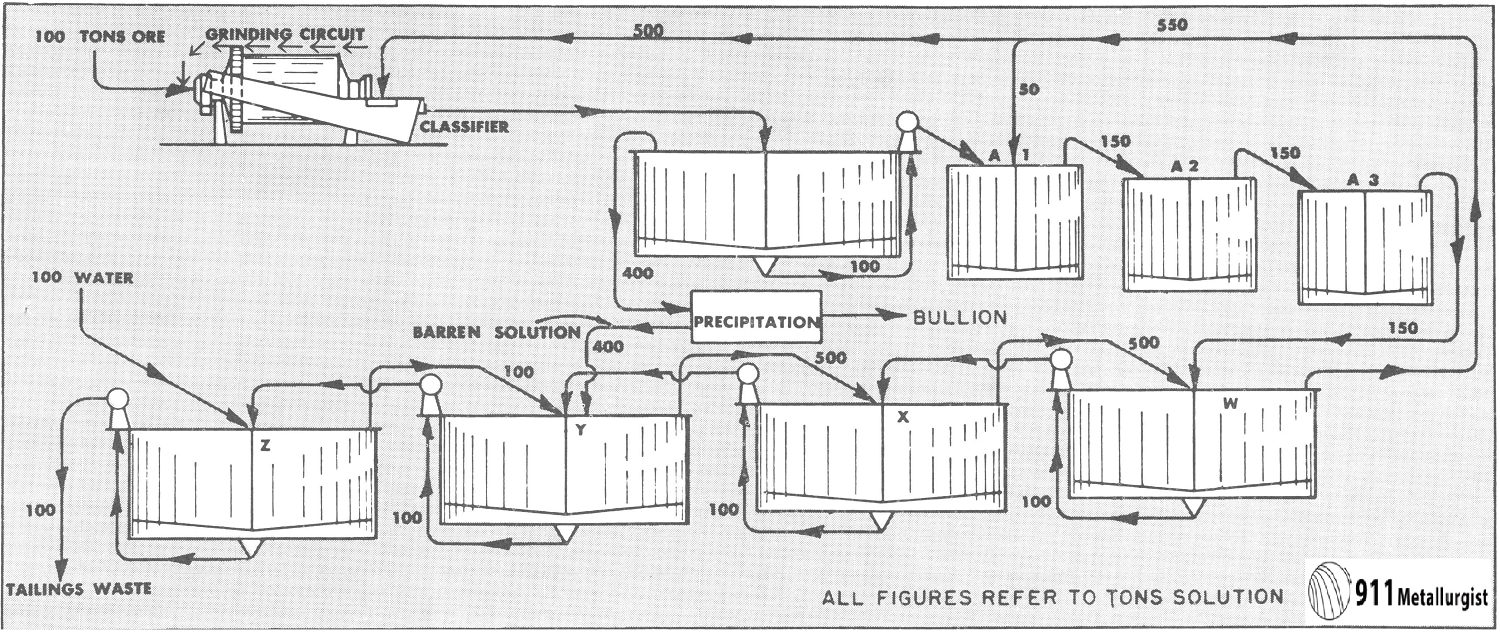### CALCULATIONS FOR DISSOLVED VALUE LOSS

To scale up these 1955 numbers, just multiply by 9X and that will approximate 2016

Conditions assumed:

1. 100 tons ore per 24 hour ground in cyanide solution.
2. All thickener underflows discharged at 50% solids.
3. \$20.00 value dissolved per ton of ore.
4. 75% dissolved in grinding circuit; balance in agitators.
5. 400 tons of solution precipitated to \$0.015/ton.
6. Pulp agitated at 40% solids (1½ to 1).
7. Let V, W, X, Y and Z represent dollar value of solution discharged from each thickener.

Equating out and into each thickener we have:

• Thickener V—100V+400V=500W+(0.75X\$20X 100 tons)
• Thickener W—100W+550W=500X+50W+(0.25X\$20X100 tons)+100V
• Thickener X—100X+500X= 100W+500Y
• Thickener Y—100Y+500Y=100Z+100X +(400X \$0.015)
• Thickener Z—100Z+100Z=100Y+100 tons water, value \$0.00

Simplifying:

1. V=W+3                                 V=\$5.00444
2. W=X+1.6                            W=\$2.00444
3. X=Y+0.32                          X=\$0.40444
4. Y=0.2Z+0.076                 Y=\$0.08444
5. 2Z=Y                                     Z=\$0.04222

To check these figures: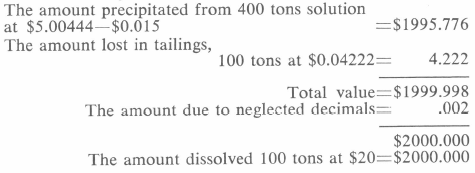From the foregoing the following results are deduced:
Assay value of pregnant solution. Value of V =\$5.00444
Assay value of discharged solution. Value of Z =\$0.04222
Loss of dissolved value per ton of ore =\$0.04222
Dissolved value saved =99.8%

With an ore of the value as used in these calculations it would be desirable to increase the volume of solution to precipitation and thereby cut down on the dissolved loss. This extra solution from thickener W would be added to the classifier overflow feeding into the primary thickener V. Only sufficient solution is added to the grinding circuit to meet the requirements of the grind necessary. It is highly desirable to cut the solution loss to less than 2 cents per ton.

Calculations from the mechanical loss of cyanide:

Assumed conditions:

1. Neglect the cyanide consumption throughout the system
2. Strength of cyanide (NaCN) solution 1.00 lb. per ton
3. Let V, W, X, Y and Z represent the strength in lbs. of NaCN solution discharged from the respective thickeners.

Equating out and into each thickener:

1. V=1.0
2. 100W+550W=50W+100V+500X
3. 100X+500X=100W+500Y
4. 100Y+500Y=100Z+400V+100X
5. 100Z+100Z=100Y+100 tons H2O at no value.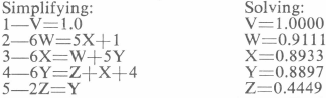Mechanical loss of cyanide per ton of ore=Z=0.4449 lbs.

While there have been certain short cut methods developed for calculation of solution values and losses, it is very important for the cyanide plant metallurgist to fully understand the application of the simultaneous equation method as presented. One such short cut method is detailed in the AIME Paper entitled “Continuous Counter Current Decantation Calculations,” by T. B. Counselman, Volume 187, February, 1950. Other sources of good information are in the “Handbook of Cyanidation,” by Hamilton and “Cyanidation Concentration of Gold and Silver Ores,” by Dorr and Bosqui.

In actual practice, the best procedure is sample the solution at various points in the system and assay for contained values. This also applies to determining the cyanide and lime strength which is done by titration methods. The assumed conditions for the algebraic calculations are never quite met in practice, for the values due to a certain extent continue to dissolve in the primary, thicken and may also continue on through to washing stages. This is particularly so with ores containing both gold and silver, for the latter usually dissolves at a slower rate.

Gold Leaching Plant for Sale

Gold Leaching Plant for Sale

Counter Current Decantation Design Formula

ccd-countercurrent-decantation
countercurrent-decantation
variables-affecting-countercurrent-decantation

Design of Counter Current Decantation in Copper Metallurgy by joseph kafumbila on Scribd

graphical-representation-of-theoretical-soluble-losses-by-ccd